Next: Computing Eigenvalues and Eigenvectors Up: Face Normalization and Recognition Previous: Typical Normalization Results

# Karhunen-Loeve Decomposition for Statistical Recognition and Detection

At this stage, we have synthesized a normalized mug-shot for each individual in a scene. The large, nonlinear variance due to pose and illumination has been eliminated and it is now possible to classify individuals by simple linear techniques. We consider the use of Karhunen-Loeve Decomposition (KL), also known as Principal Component Analysis (PCA) on the intensity images . This technique is traditionally used in statistical signal detection and estimation theory and has been adapted to compression and recognition.

Each intensity image is converted into a (raster) vector form. Note that this purely intensity-based coding of the image is not necessarily ideal for the application of KL-decomposition.4.3The normalized mug-shots in a database are represented by an ensemble of vectors,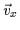. Since the mug-shot images are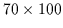pixels, these vectors are 7000-tuples or 7000 element vectors. A total of 338 such mug-shot images are acquired and converted into vector form. The ensemble of vectorsis assumed to have a multi-variate Gaussian distribution since faces form a dense cluster in the large 7000-dimensional image space. We wish to produce an efficient decomposition which takes advantage of the redundancies or correlations in this data. Linear variance analysis makes it possible to generate a new basis which spans a lower dimensional subspace than the original 7000-dimensional space and simultaneously accounts optimally for the variance in the ensemble. PCA generates this small set of basis vectors forming this subspace whose linear combination gives the ideal approximation to the original vectors in the ensemble. Furthermore, the new basis exclusively spans intra-face and inter-face variations, permitting Euclidean distance measures in the sub-space to exclusively measure changes in identity and expression. Thus, we can use simple distance measurements in the subspace as a classifier for recognition.

We shall perform KL decomposition on an n=7000 dimensional subspace with N=338 training vectors. Some of thesevectors are shown in Figure. We begin by computing the mean,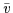, of the 338 vectors using Equationwhich generates Figure: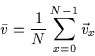(4.24)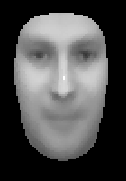We then generate deviation vectors,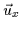, as in Equationand arrange them in a dataset matrix, D, as in Equation. The dimensions of D are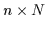: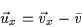(4.25)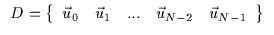(4.26)

The covariance matrix C of our dataset is computed and its eigenvectors will form the orthonormal basis which optimally spans the subspace of the data (human faces). There exist two ways of computing and manipulating C which yield the same result yet with different efficiencies . We begin by considering C generated using Equation.

 C = DT D (4.27)

We can compute the n eigenvalues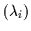and eigenvectors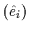of this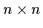symmetric matrix. The eigenvectors and their corresponding eigenvalues are ranked such that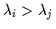for i<j. Mercer's theorem  can be used to obtain Equation: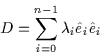(4.28)

Note that the magnitude of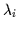is equal to the variance in the dataset spanned by its corresponding eigenvector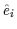(see Equation):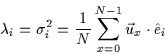(4.29)

It then follows that any vector,, in the dataset, D, can be optimally approximated as in Equation. Thus, the n-dimensional face deviation vector can be re-defined as a linear combination of eigenvectors determined by M coefficients denoted by cxi (computed using Equation. M is the number of eigenvectors used to approximate the original vector. The larger the value of M, the more eigenvectors are used in the approximation and, consequently, the more accurate it becomes. M can be reduced allowing more efficient storage of each face. However, the quality of the approximation degrades gradually as fewer eigenvectors and coefficients are used in the linear combination :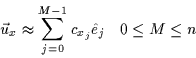(4.30)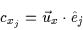(4.31)

Alternatively, we can obtain the same eigenvalues and eigenvectors from C', another symmetric covariance matrix, which is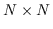. C' is defined in Equation:

 C' = D DT (4.32)

This equation yields N eigenvalues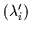and eigenvectors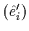. These re also ranked such that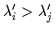for i<j. It is possible to then directly compute the first N eigenvalues and eigenvectors of C, as given by Equation: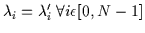(4.33)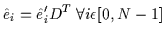(4.34)

We shall now describe the covariance matrix we select as well as actual method used to extract the eigenvalues and eigenvectors.Next: Computing Eigenvalues and Eigenvectors Up: Face Normalization and Recognition Previous: Typical Normalization Results
Tony Jebara
2000-06-23